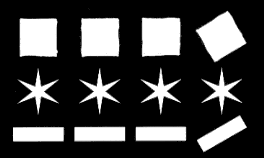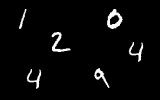We have a few task to implement in this course:

• Implement a simple object classifier,
• implement a backpropagation neural network classifier,
• use a deep learning framework to implement a backpropagation neural network classifier,
• compute a well know object heatures that can be used in a classifier.

## Evaluation - how can you earn a credit

During the exercises, we will cover some topic of digital image processing. Your task is complete and handover them for final grading. We'll use the OpenCV library in our implementation.

 Task Points Thresholding 1 Indexing 1 Computing moments 2 Features from moments 2 Classficition using etalons 3 Classification using k-means 4 Own neural network 6 Neural network in PyTorch 2 Deep neural network for number classification 3 Histogram of Oriented Gradients 3 Classification using HOG 3 SLIC 4 Background subtraction (MOG) 6

## Exercise 1

We'll implement image segmentation using thresholding and indexing of objects on the following input image:Text describing the content of the exercise is available in [PDF] or as a web presentation.

Template projects for different development environments and operating systems that you can use to implement exercises:

If you use Linux and VSCode, first, install these two VSCode extensions first:

```        code --install-extension ms-vscode.cpptools
code --install-extension ms-vscode.cmake-tools
```

You can read an explanatory text that describes the first exercise in detail. Basic operations with images and datatypes that you'll need to know are described.

## Exercise 2

We'll implement a simple features computation.

We'll implement features computation: features_02 [PDF] or in a web presentation.

## Exercise 3

We'll implement saving the ethalons features and use this features to classify objects in a new image. A description is provided in this document.

## Exercise 4

We'll implemente k-means algorithm for simple clustering of extracted features.

## Exercise 5

We'll use a back propagation neural network as a classifier. See text about back propagation neral network for details. The basic structure of a back propagation neural network is in the following file: bpnn.zip

## Exercise 6

We'll use a back propagation neural network as a classifier.

## Exercise 7

We'll look at the motion detection based on background modeling usign Gaussian Mixture Model (GMM). The text that describes the method is available here: mog.pdf (in Czech, author: Tomáš Fabián)

You can also go through an introduction to motion detection in English: Understading Background Mixture Models for Foreground Segmentation.

A video that we can use for our experiments is also available: dt_passat.mpg

## Exercise 8

Finish up the implementation of MOG from the previous exercise.

## Exercise 9

We'll implement a SLIC segmentation. The image from the previous document is here.

## Exercise 10

We'll implement a back propagation neural network as a classifier in the PyTorch deep learning framwrork. Use this Jupyter Notebook as a stub.

We'll continue implementing a back propagation neural network as a classifier.

## Exercise 11

We'll implement a sliding window for numbers detection and classificatin using the PyTorch deep learning framwrork. Use this Jupyter Notebook as a stub.

## Exercise 12

We'll implement the Histogram of Oriented Gradients for object classification/detection.

You can test your implementation of the following image:## Exercise 13

Use HOG features to detect and classify numbers in numbers image.
Use sliding window technique to detect each number. Use a neural network (your own implementation, PyTorch or a network in the OpenCV). Use images from MNIST dataset to train and test the network that can be found here.Final exercise.